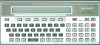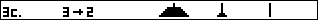##### Sharp PC-1500 : HANOI TOWERS game

"Hanoi towers" for Sharp PC-1500 or PC-1500A.
Author : ? (**)
Published in "La revue des Sharpentiers" #8.
BASIC program - 1130 bytes.RUN or DEF SPACE

Game: A classic puzzle. You must move all the discs from left to right, one at a time, using a minimum number of moves. A disc can be moved only onto a larger one.

Keys:
\$ % &

(*) This software is made available for documentation purpose only, since the PC-1500 is now an obsolete computer. This is not a commercial site. If you own a copyright on this program and do not want it to be available from this site, please inform me and I will remove it.

(**) Anybody out there can provide the name of the author of this program ? Thanks.

BASIC program.

7 "HANOI TOWERS
8 "KEYS : \$%&
9 "SHARPENTIERS #8
10 " "CLEAR :DIM B(7,3):E=85,U=24,B(0,1)=99,B(0,2)=99,B(0,3)=99
20 PAUSE " *** HANOI TOWERS ***":INPUT "How many discs (1-7)? ";Q:Q=INT Q:IF Q<1OR Q>7THEN 20
30 B=Q,F=2*Q+1:FOR I=1TO Q:B(I,1)=F,F=F-2:NEXT I
40 CLS :WAIT 0:FOR I=0TO Q-1:GCURSOR E-Q+I:V=V+2^(6-I):GPRINT V:NEXT I
50 FOR I=7-QTO 6:GCURSOR E+I-6+Q:GPRINT V:V=V-2^I:NEXT I:GCURSOR E:GPRINT &7F:GCURSOR E+U:GPRINT &7F:GCURSOR E+2*U:GPRINT &7F
60 S=ASC INKEY\$ :IF S<20OR S>22THEN 60
70 Z=S-19:IF @(Z+1)=0THEN 110
80 BEEP 1:K=30,H=Z:GOSUB 200:GPRINT "001010103810000006520E00"
90 S=ASC INKEY\$ :IF S<20OR S>22THEN 90
100 X=S-19:IF Z<>XAND B(@(Z+1),Z)<B(@(X+1),X)THEN 120
110 GCURSOR 30:GPRINT "06520E00":BEEP 3:GOTO 60
120 BEEP 1:K=42,H=X:GOSUB 200
130 L=E+U*(Z-1)-INT (B(@(Z+1),Z)/2),T=L+U*(X-Z)
140 FOR M=0TO B(@(Z+1),Z)-1:N=L+M:GCURSOR N:GPRINT POINT NAND (127-2^(7-@(Z+1))):GCURSOR E+U*(Z-1)
150 GPRINT &7F:R=2^(6-@(X+1)),N=M+T:GCURSOR N:GPRINT POINT NOR R:NEXT M
160 B(@(X+1)+1,X)=B(@(Z+1),Z),B(@(Z+1),Z)=0
170 @(Z+1)=@(Z+1)-1,@(X+1)=@(X+1)+1,A=A+1
180 IF D=QCLS :WAIT :PRINT "BRAVO !!!";USING "####";A;" MOVES.":END
190 K=0,W\$=STR\$ A:FOR I=1TO LEN W\$:H=VAL (MID\$ (W\$,I,1)):GOSUB 200:K=K+4:NEXT I:GPRINT "7848480040":GOTO 60
200 GCURSOR K:RESTORE 210+10*H:READ A\$:GPRINT A\$,:RETURN
210 DATA "7C447C
220 DATA "487C40
230 DATA "74545C
240 DATA "54547C
250 DATA "1C7010
260 DATA "5C5474
270 DATA "7C5474
280 DATA "04047C
290 DATA "7C547C
300 DATA "5C547C